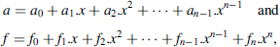## 8.4 OPERATIONS IN GF(pn)

If f is an irreducible polynomial then Zp[x]/f(x) is the Galois field GF(pn), so that every nonzero polynomial a(x) has a multiplicative inverse a−1(x). Given two polynomialsa variant of the extended Euclidean algorithm (Chapter 2, Section 2.1.2) allows the expression of the greatest common divider of f and a in the formIn particular, if gcd(f, a) = 1 then a−1(x) = b(x) mod f.

The following formal algorithm, in which degree(a) returns the degree of a and swap(a, b) interchanges a and b, computes z(x) = a−1(x).

Algorithm 8.23

u:=f; v:=a; c:=0; e:=1; m:=degree(u); t:=degree(v); if t=0 then result:=(v(0))−1; else while t>0 loop if m<t then swap(u,v); swap(c,e); swap(m,t); q:=u(m)*(v(t))-1*xm−t; r:=u-(v*q); cc:=c-(e*q); u:=v; v:=r; c:=e; e:=cc; m:=t; t:=deg(v); end loop; z:=e*(v(0))-1; end if;

Example 8.7As p = 2, Algorithm 8.23 can be simplified; in particular, u(m) = υ−1(t) = υ(0) = 1.

Compute a−1(x):Effectively,In order to execute Algorithm 8.23, the ...

Get Synthesis of Arithmetic Circuits: FPGA, ASIC and Embedded Systems now with the O’Reilly learning platform.

O’Reilly members experience books, live events, courses curated by job role, and more from O’Reilly and nearly 200 top publishers.